Next: Magnetic Field Up: C.1 One Homogeneous Planar Previous: C.1 One Homogeneous Planar

### Electric Field

We assume that a homogeneous plane wave coming from a certain direction k0 = (kx  ky  k0, z)T strikes onto a planar homogeneous layer l. Within the layer the electric field consists of two plane waves traveling in opposite directions kl+ and kl-. This situation is schematically illustrated in Figure C.1. The electric phasor El(x) is thus written as(C.1)

whereby El+ and El- are the wave amplitudes traveling downwards and upwards the layer. The two wavevectors are given by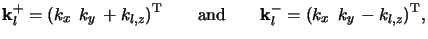(C.2)

and the vertical wavevector component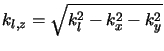(C.3)

depends on the wavenumber kl = k0nl of the layer material with refractive index nl.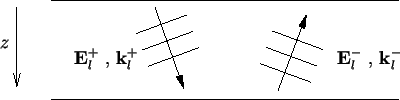Due to the transverseness of the plane waves the vertical amplitude components can be expressed by the lateral ones, i.e.,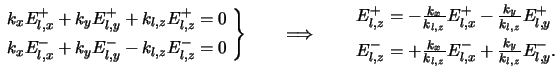(C.4)

Hence it suffices to study only the lateral field components of (C.1) given by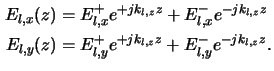In these two equations El, x(z) and El, y(z) refer to the z-dependent part of the electric field phasor. As can be seen from (C.1) the complete phasor is obtained by multiplication with the exponential factor exp(j(kxx + kyy)), which describes the lateral dependence.Next: Magnetic Field Up: C.1 One Homogeneous Planar Previous: C.1 One Homogeneous Planar
Heinrich Kirchauer, Institute for Microelectronics, TU Vienna
1998-04-17